Published: 30 June 2020

# Qualities of a vibrator, the elastic element of which has different coefficients of stiffness in the two intervals of displacements, in a separate case

K. Ragulskis1
B. Spruogis2
M. Bogdevičius3
A. Matuliauskas4
V. Mištinas5
L. Ragulskis6
1Kaunas University of Technology, K. Donelaičio Str. 73, LT-44249, Kaunas, Lithuania
2, 3, 4, 5Department of Mobile Machinery and Railway Transport, Faculty of Transport Engineering, Vilnius Gediminas Technical University, Plytinės Str. 27, LT-10105, Vilnius, Lithuania
6Department of Systems Analysis, Faculty of Informatics, Vytautas Magnus University, Vileikos Str. 8, LT-44404, Kauna, Lithuania
Corresponding Author:
K. Ragulskis
Views 63

#### Abstract

Generators of mechanical vibrations with nonlinear stiffness of the elastic element in the conservative case are investigated. It is shown that the vibrator of this type generates several harmonic components and that the number of those multiple harmonics can be controlled. One period of motion is investigated. The presented analytical relationships and numerically obtained graphical relationships reveal the qualities of the system and enable to choose the desirable parameters of motions. The performed investigation of this system showed that eigenvibrations take place with linear spectrums when the system in separate intervals consists from two linear parts. This takes place in the case when the border of difference of coefficients of stiffness is located in the position of equilibrium of the system. In both intervals vibrations by separate partial frequencies take place and general motion depends on the eigenfrequency of the whole system. Of course the latter frequency depends on both partial frequencies. General motion of the whole system takes place according to the infinite linear spectrum of eigenfrequencies. All this enables to create enhanced vibrators by using those qualities and to use them in technologies.

## 1. Introduction

In a number of publications basis of the theory of nonlinear mechanical generators of vibrations were investigated as well as their multi valued steady state regimes. The distinctive feature of this research work is in the fact that they can generate several frequencies under excitation of a single harmonic as well as ensure stable operation in the resonance zones.

This paper is based on the investigations of essentially nonlinear systems and their dynamics presented in a book by Ragulskienė V. Vibro-Shock Systems (Theory and Applications). Vilnius: Mintis, 1974, (in Russian), where change of the jump type in the characteristic of impact velocity which takes place at the point of transition between soft and hard excitations of vibrations is seen. But the essential features are not revealed. This quality was also observed when exciting vibrations of vibromotors in a book by Ragulskis K., Bansevičius R., Barauskas R., Kulvietis G. Vibromotors for Precision Microrobots. New York: Hemisphere, 1987. Here also attention was not directed to this quality. Papers presented in the references were useful for investigations. Nonlinear vibrating systems and their resonances are analysed in . Periodic and transient excitations and the dynamics of impacts are investigated in . Periodic nonlinear systems as well as their stabilization are analysed in . Mechanical systems when impacts take place are investigated in . Mechanical systems, impacts and their periodic orbits are described in . Nonlinear vibro-impact energy sink is analysed in . Impact of particle with a wall is investigated in . Determination of frequencies of a mechanical system is analysed in . Mechanism with a pendulum is described and investigated in . Vibrations of a model having piecewise linearity are analysed in . Resonant zones of a dynamical system are investigated in . Investigation of the Sommerfeld effect is performed in . Analysis of isolated resonances of a dynamical system is presented in .

Several systems were investigated in which subsystems with different nonlinearities have a point of transition and when this point in the static positions of both subsystems coincides, for example . In this paper the investigation is presented when the quality of this type takes place and the independence of eigenfrequencies from the amplitudes of motions is determined and motion takes place with infinite spectrum of frequencies.

In this paper the system consisting from two subsystems the static positions of equilibrium of which coincide is investigated. The motions are investigated analytically – graphically and they indicate the linearity of spectrums with infinite number of frequencies.

The investigated system is described by the equations of the following type:

1
$\stackrel{¨}{x}+\left({p}^{2}+{p}_{0}^{2}\right)x=f\mathrm{s}\mathrm{i}\mathrm{n}\left(\omega t+\phi \right),\mathrm{}\mathrm{}\mathrm{}\mathrm{}\mathrm{}x={x}_{0},$
2
$\stackrel{¨}{x}+{p}^{2}x=f\mathrm{s}\mathrm{i}\mathrm{n}\left(\omega t+\phi \right),\mathrm{}\mathrm{}\mathrm{}\mathrm{}\mathrm{}x<{x}_{0},$
3
${p}_{0}^{2}{x}_{0}=0,\mathrm{}\mathrm{}\mathrm{}\mathrm{}\mathrm{}x<{x}_{0},$

where $p=\sqrt{C/m}$, ${p}_{0}=\sqrt{{C}_{0}/m}$, $f=F/m$, $\omega$ and $\phi$ are the frequency and phase of excitation respectively, $p$ and $p+{p}_{0}$ are partial eigenfrequencies of the system.

## 2. Conservative system

Characteristics of the system are determined analytically – numerically when $f=0.$

### 2.1. Motion of the system in the first interval

Investigation of motion of the system in the interval:

4
$t\in \left[0,{T}_{0}\right],\mathrm{}\mathrm{}\mathrm{}\mathrm{}\mathrm{}x={x}_{0},$

is performed.

The following conditions of motion are assumed when:

5
$\begin{array}{l}t=0,\mathrm{}\mathrm{}\mathrm{}\mathrm{}\mathrm{}\mathrm{}x={x}_{0}=0,\mathrm{}\mathrm{}\mathrm{}\mathrm{}\mathrm{}\mathrm{}\stackrel{˙}{x}={\stackrel{˙}{x}}^{-},\\ t={T}_{0},\mathrm{}\mathrm{}\mathrm{}\mathrm{}\mathrm{}\mathrm{}x={x}_{0}=0,\mathrm{}\mathrm{}\mathrm{}\mathrm{}\mathrm{}\mathrm{}\stackrel{˙}{x}=-{\stackrel{˙}{x}}^{-}.\end{array}$

From the Eq. (1) by taking into account Eqs. (4) and (5) it is obtained:

6
$x=\frac{{\stackrel{˙}{x}}^{-}}{\sqrt{{p}^{2}+{p}_{0}^{2}}}\mathrm{s}\mathrm{i}\mathrm{n}\sqrt{{p}^{2}+{p}_{0}^{2}}t,$
7
$\stackrel{˙}{x}={\stackrel{˙}{x}}^{-}\mathrm{c}\mathrm{o}\mathrm{s}\sqrt{{p}^{2}+{p}_{0}^{2}}t,$
8
$\stackrel{¨}{x}=-{\stackrel{˙}{x}}^{-}\sqrt{{p}^{2}+{p}_{0}^{2}}\mathrm{s}\mathrm{i}\mathrm{n}\sqrt{{p}^{2}+{p}_{0}^{2}}t,$
9
$\stackrel{˙}{x}\stackrel{¨}{x}=-0.5{\left({\stackrel{˙}{x}}^{-}\right)}^{2}\sqrt{{p}^{2}+{p}_{0}^{2}}\mathrm{s}\mathrm{i}\mathrm{n}2\sqrt{{p}^{2}+{p}_{0}^{2}}t.$

From the Eq. (7) by taking into account Eqs. (5) it is obtained:

10
${T}_{0}=\frac{\pi }{\sqrt{{p}^{2}+{p}_{0}^{2}}}.$

### 2.2. Motion of the system in the second interval

Investigation of motion of the system in the interval:

11
$t\in \left[{T}_{a},\stackrel{-}{T}\right]=\left[{T}_{a},\frac{2\pi }{\stackrel{-}{\omega }}\right],\mathrm{}\mathrm{}\mathrm{}\mathrm{}\mathrm{}\mathrm{}x\le {x}_{0},$

where $\stackrel{-}{T}$ and $\stackrel{-}{\omega }$ are the eigenperiod and eigenfrequency of the system respectively, is performed.

At:

12
$t=\stackrel{-}{T},\mathrm{}\mathrm{}\mathrm{}\mathrm{}\mathrm{}\mathrm{}x={x}_{0}=0,\mathrm{}\mathrm{}\mathrm{}\mathrm{}\mathrm{}\mathrm{}\stackrel{˙}{x}={\stackrel{˙}{x}}^{-}.$

From the Eq. (3) by taking into account Eq. (5) it is obtained:

13
$x=-\frac{{\stackrel{˙}{x}}^{-}}{p}\mathrm{s}\mathrm{i}\mathrm{n}p\left(t-{T}_{0}\right),$
14
$\stackrel{˙}{x}=-{\stackrel{˙}{x}}^{-}\mathrm{c}\mathrm{o}\mathrm{s}p\left(t-{T}_{0}\right),$
15
$\stackrel{¨}{x}={\stackrel{˙}{x}}^{-}p\mathrm{s}\mathrm{i}\mathrm{n}p\left(t-{T}_{0}\right),$
16
$\stackrel{˙}{x}\stackrel{¨}{x}=-0.5{\left({\stackrel{˙}{x}}^{-}\right)}^{2}p\mathrm{s}\mathrm{i}\mathrm{n}2p\left(t-{T}_{0}\right).$

From the Eq. (13) by taking into account Eqs. (10) and (12) it is obtained:

17
$\stackrel{-}{T}=\frac{\pi }{\sqrt{{p}^{2}+{p}_{0}^{2}}}+\frac{\pi }{p}\mathrm{}\mathrm{}\mathrm{}\mathrm{o}\mathrm{r}\mathrm{}\mathrm{}\mathrm{}\mathrm{}\stackrel{-}{T}=\frac{\pi }{p}\left[1+\frac{1}{\sqrt{1+{\left(\frac{{p}_{0}}{p}\right)}^{2}}}\right].$

By taking into account that $\stackrel{-}{T}=2\pi /\stackrel{-}{\omega }$ and on the basis of Eq. (16) it is obtained:

18
$\stackrel{-}{\omega }=2p\frac{\sqrt{1+{\left(\frac{{p}_{0}}{p}\right)}^{2}}}{\sqrt{1+{\left(\frac{{p}_{0}}{p}\right)}^{2}}+1},$

which is used for the determination of the eigenfrequency as a function of the parameters of the investigated system.

### 2.3. Characteristics of the system

Dynamical characteristics of the system $x$, $\stackrel{˙}{x}$, $\stackrel{¨}{x}$, $\stackrel{˙}{x}\stackrel{¨}{x}$ are expanded into the Fourier series with respect to the eigenfrequency.

As an example the expansion of the characteristics of displacement is presented. According to the Eqs. (5) and (13):

19
$x=\frac{{\stackrel{˙}{x}}^{-}}{p}\left\{\begin{array}{l}\frac{1}{\sqrt{1+{\left(\frac{{p}_{0}}{p}\right)}^{2}}}\mathrm{s}\mathrm{i}\mathrm{n}\sqrt{1+{\left(\frac{{p}_{0}}{p}\right)}^{2}}t,\mathrm{}\mathrm{}\mathrm{}\mathrm{}\mathrm{}\mathrm{}0\le t\le {\stackrel{-}{T}}_{0}=\frac{\pi }{p\sqrt{1+\left(\frac{{p}_{0}}{p}\right)}},\\ -\mathrm{s}\mathrm{i}\mathrm{n}p\left(t-{T}_{0}\right),\mathrm{}\mathrm{}\mathrm{}\mathrm{}\mathrm{}\mathrm{}{T}_{0}\le t\le \stackrel{-}{T},\end{array}\right\$

it is obtained:

20
$x=\frac{{\stackrel{˙}{x}}^{-}}{p}\sum _{n=0}^{\mathrm{\infty }}\left({a}_{n}\mathrm{s}\mathrm{i}\mathrm{n}n\stackrel{-}{\omega }t+{b}_{n}\mathrm{s}\mathrm{i}\mathrm{n}n\stackrel{-}{\omega }t\right),$

where ${a}_{n}$ and ${b}_{n}$ depend only from $p$ and ${p}_{0}/p.$

In the same way other characteristics are also expanded into the Fourier series:

21
$\stackrel{˙}{x}={\stackrel{˙}{x}}^{-}\left\{\begin{array}{l}\mathrm{c}\mathrm{o}\mathrm{s}p\sqrt{1+{\left(\frac{{p}_{0}}{p}\right)}^{2}}t,\mathrm{}\mathrm{}\mathrm{}\mathrm{}\mathrm{}\mathrm{}0\le t\le {T}_{0},\\ -\mathrm{c}\mathrm{o}\mathrm{s}p\left(t-{T}_{0}\right),\mathrm{}\mathrm{}\mathrm{}\mathrm{}\mathrm{}\mathrm{}\mathrm{}{T}_{0}\le t\le \stackrel{-}{T},\end{array}\right\$
22
$\stackrel{¨}{x}={\stackrel{˙}{x}}^{-}p\left\{\begin{array}{l}-\sqrt{1+{\left(\frac{{p}_{0}}{p}\right)}^{2}}\mathrm{s}\mathrm{i}\mathrm{n}p\sqrt{1+{\left(\frac{{p}_{0}}{p}\right)}^{2}}t,\mathrm{}\mathrm{}\mathrm{}\mathrm{}\mathrm{}\mathrm{}0\le t\le {T}_{0},\\ \mathrm{s}\mathrm{i}\mathrm{n}p\left(t-{T}_{0}\right),\mathrm{}\mathrm{}\mathrm{}\mathrm{}\mathrm{}{T}_{0}
23
$\stackrel{˙}{x}\stackrel{¨}{x}=-0.5{\left({\stackrel{˙}{x}}^{-}\right)}^{2}p\left\{\begin{array}{l}\sqrt{{p}^{2}+\frac{{p}_{0}}{p}}\mathrm{s}\mathrm{i}\mathrm{n}2p\sqrt{p+{\left(\frac{{p}_{0}}{p}\right)}^{2}}t,\mathrm{}\mathrm{}\mathrm{}\mathrm{}\mathrm{}\mathrm{}0\le t\le {T}_{0},\\ \mathrm{s}\mathrm{i}\mathrm{n}2p\left(t-{T}_{0}\right),\mathrm{}\mathrm{}\mathrm{}\mathrm{}\mathrm{}\mathrm{}{T}_{0}

According to the Eqs. (19) and Eqs. (21-23) their investigation is performed by obtaining graphical relationships $\stackrel{˙}{x}=\stackrel{˙}{x}\left(x\right)$, $\stackrel{¨}{x}=\stackrel{¨}{x}\left(\stackrel{˙}{x}\right)$, $\stackrel{˙}{x}\stackrel{¨}{x}=\stackrel{˙}{x}\stackrel{¨}{x}\left(x\right)$; $x$, $\stackrel{˙}{x}$, $\stackrel{¨}{x}$, $\stackrel{˙}{x}\stackrel{¨}{x}$ as functions of time in the period $\stackrel{-}{T}$ and also the coefficients of their expansion into the Fourier series, that is the graphical representations of eigenfrequencies.

## 3. Results of investigations of dynamics of the conservative system

Graphical results of investigation of dynamics of the system when ${p}^{2}=1$, ${p}_{0}^{2}=1$ are presented in Fig. 1.

Graphical results of investigation of dynamics of the system when ${p}^{2}=$ 1, ${p}_{0}^{2}=$ 4 are presented in Fig. 2.

Graphical results of investigation of dynamics of the system when ${p}^{2}=\text{4}$, ${p}_{0}^{2}=\text{1}$ are presented in Fig. 3.

Amplitude frequency characteristics when ${p}^{2}=$ 1, ${p}_{0}^{2}=$ 1 are presented in Fig. 4.

Amplitude frequency characteristics when ${p}^{2}=$ 1, ${p}_{0}^{2}=$ 4 are presented in Fig. 5.

Amplitude frequency characteristics when ${p}^{2}=$ 4, ${p}_{0}^{2}=$ 1 are presented in Fig. 6.

Fig. 1Dynamics of the conservative system when p2= 1, p02= 1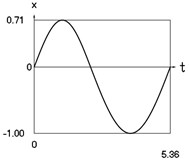a) Displacement as function of time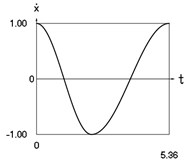b) Velocity as function of time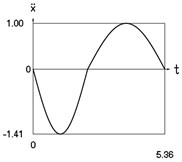c) Acceleration as function of time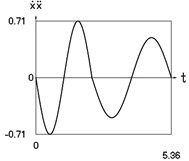d) Velocity multiplied by acceleration as function of time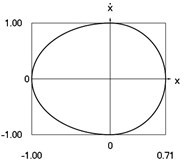e) Phase trajectory: velocity as function of displacement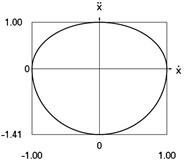f) Phase trajectory: acceleration as function of velocity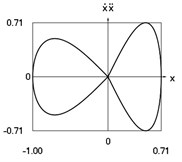g) Phase trajectory: velocity multiplied by acceleration as function of displacement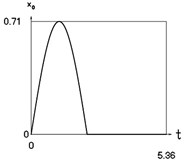h) Another displacement as function of time

Fig. 2Dynamics of the conservative system when p2= 1, p02= 4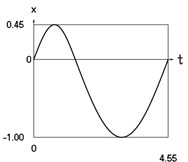a) Displacement as function of time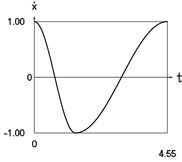b) Velocity as function of time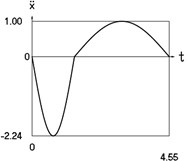c) Acceleration as function of time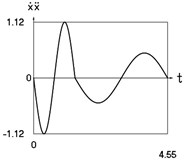d) Velocity multiplied by acceleration as function of time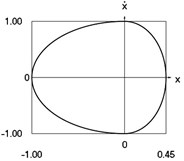e) Phase trajectory: velocity as function of displacement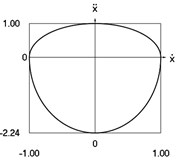f) Phase trajectory: acceleration as function of velocity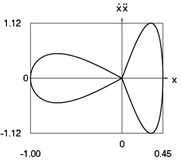g) Phase trajectory: velocity multiplied by acceleration as function of displacement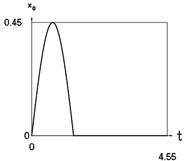h) Another displacement as function of time

Fig. 3Dynamics of the conservative system when p2= 4, p02= 1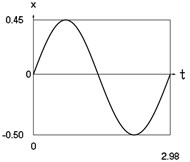a) Displacement as function of time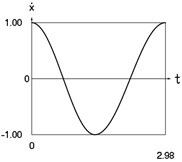b) Velocity as function of time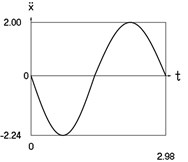c) Acceleration as function of time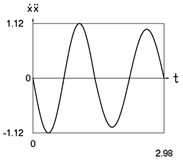d) Velocity multiplied by acceleration as function of time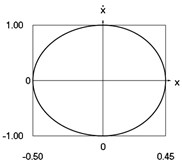e) Phase trajectory: velocity as function of displacement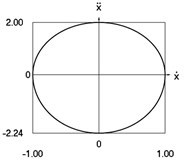f) Phase trajectory: acceleration as function of velocity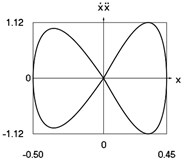g) Phase trajectory: velocity multiplied by acceleration as function of displacement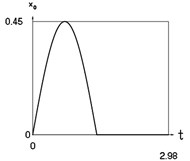h) Another displacement as function of time

Fig. 4Amplitude frequency characteristics (constant part and first three harmonics) when p2= 1, p02= 1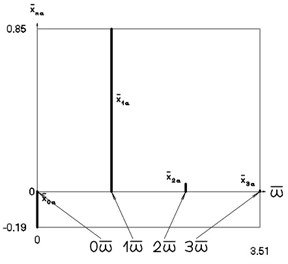a) Displacement frequency characteristic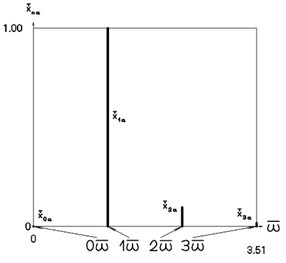b) Velocity frequency characteristic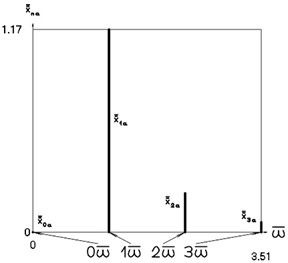c) Acceleration frequency characteristic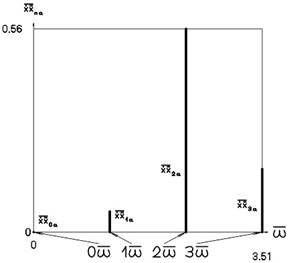d) Velocity multiplied by acceleration frequency characteristic

Fig. 5Amplitude frequency characteristics (constant part and first three harmonics) when p2= 1, p02= 4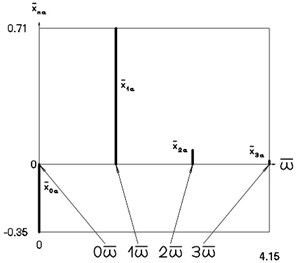a) Displacement frequency characteristic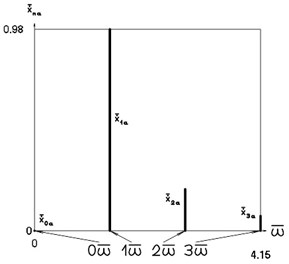b) Velocity frequency characteristic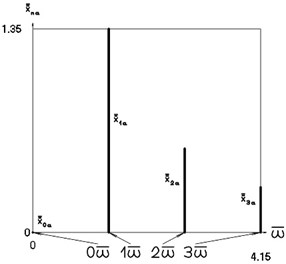c) Acceleration frequency characteristic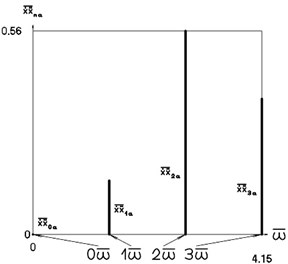d) Velocity multiplied by acceleration frequency characteristic

Fig. 6Amplitude frequency characteristics (constant part and first three harmonics) when p2= 4, p02= 1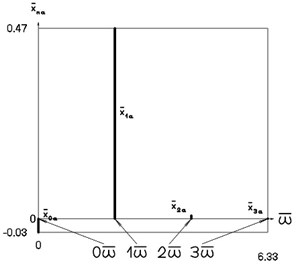a) Displacement frequency characteristic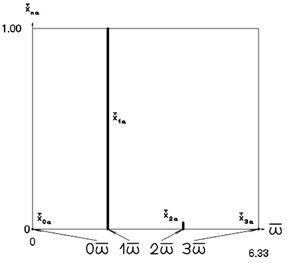b) Velocity frequency characteristic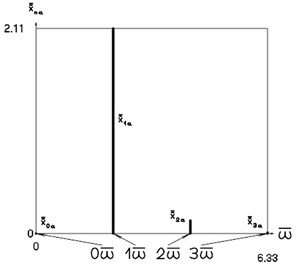c) Acceleration frequency characteristic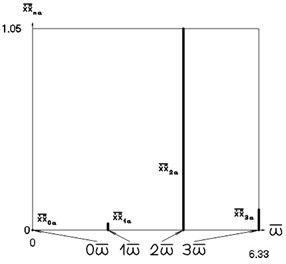d) Velocity multiplied by acceleration frequency characteristic

## 4. Conclusions

General nonlinear model of the vibrator consists from two linear parts the coefficients of stiffness of the elastic element of which have different values and the positions of static equilibrium of the general system as well as positions of static equilibrium of both constituent parts are mutually equal. It is determined that the spectrum of eigenfrequencies of the general system is infinite and that values of frequencies do not depend from the amplitude of excitation. Vibrators of this type are effective, because in the resonance zones they do not have multivalued steady state regimes. The investigations were performed by using analytical – numerical methods and they revealed the qualities of the general system.

One period of motion of the conservative system is investigated. Results of investigation of dynamics of the conservative vibrating system for various parameters of the system are presented. Phase trajectories and amplitude frequency characteristics are used for representation of qualities of the system.

The presented analytical relationships and numerically obtained graphical relationships reveal the qualities of the system and enable to choose the desirable parameters of motions.